﻿ p-Huber损失函数及其鲁棒性研究

p-Huber损失函数及其鲁棒性研究p-Huber Loss Functions and Its Robustness

Abstract: The data is often contaminated by outliers because of the complexity of the real data in the real applications. Hence it is getting more and more important to invent some statistical machine learning algorithms that are robust to outliers. In this paper, we propose a robust and non-convex p-Huber loss function based on the Huber loss. In the numerical analysis, the fitting effect of regression learning algorithm based on p-Huber loss and regression algorithm based on L1 loss, Huber loss and MCCR loss are compared. The numerical results show that the p-Huber loss function outperforms all of other common loss functions mentioned in the paper when there are outliers in the data.

Abstract:

1. 引言

$Y={f}^{\ast }\left(X\right)+\epsilon$$Ε\left(\epsilon |X=x\right)=0$(1.1)

${\varphi }^{\text{L2}}\left(y,f\left(x\right)\right)={\left(y-f\left(x\right)\right)}^{2}$(1.2)

${\varphi }^{\text{L1}}\left(y,f\left(x\right)\right)=|y-f\left(x\right)|$(1.3)

L1损失比L2损失更具鲁棒性，但它的中心点是折点，因此不能求导，从而不易于求解。

P. J. Huber等  在1964年提出了Huber损失函数，如图1(c)，其定义如下：

${\varphi }^{\text{Huber}}\left(y,f\left(x\right)\right)=\left\{\begin{array}{l}0.5{\left(y-f\left(x\right)\right)}^{2},\text{\hspace{0.17em}}\text{\hspace{0.17em}}\text{\hspace{0.17em}}\text{\hspace{0.17em}}\text{\hspace{0.17em}}\text{\hspace{0.17em}}\text{\hspace{0.17em}}\text{\hspace{0.17em}}\text{ }\text{\hspace{0.17em}}|y-f\left(x\right)|<\delta ,\\ \delta |y-f\left(x\right)|-0.5{\delta }^{2},\text{\hspace{0.17em}}\text{\hspace{0.17em}}\text{\hspace{0.17em}}\text{\hspace{0.17em}}|y-f\left(x\right)|\ge \delta ,\end{array}$ (1.4)

${\varphi }^{\text{MCCR}}\left(y,f\left(x\right)\right)={\sigma }^{2}\left(1-{\text{e}}^{\frac{{\left(y-f\left(x\right)\right)}^{2}}{{\sigma }^{2}}}\right)$(1.5)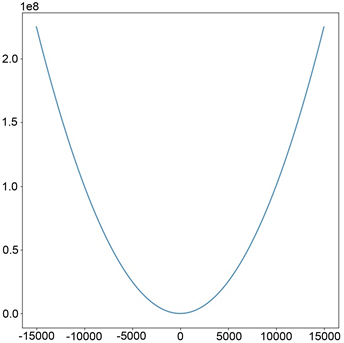(a)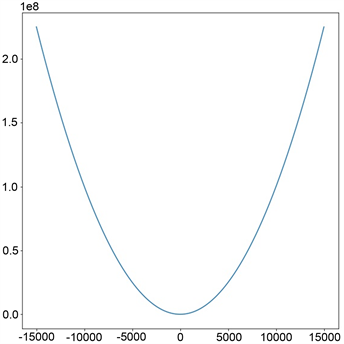(b)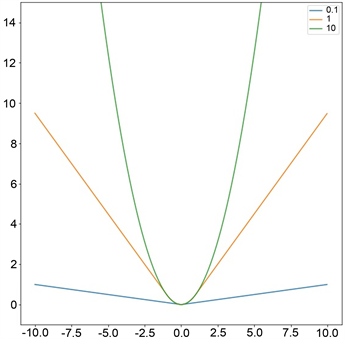(c)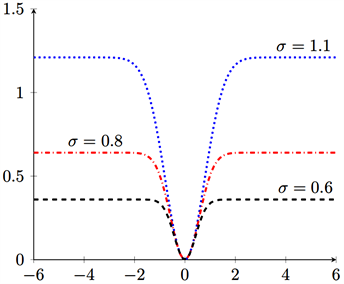(d)

Figure 1. Diagram of four loss functions

2. p-Huber损失函数及回归算法

2.1. p-Huber损失函数

${\varphi }^{p\text{-Huber}}\left(y,f\left(x\right)\right)=\left\{\begin{array}{l}{\left(y-f\left(x\right)\right)}^{2},\text{\hspace{0.17em}}\text{\hspace{0.17em}}\text{\hspace{0.17em}}\text{\hspace{0.17em}}\text{\hspace{0.17em}}\text{\hspace{0.17em}}\text{\hspace{0.17em}}\text{\hspace{0.17em}}\text{\hspace{0.17em}}\text{\hspace{0.17em}}\text{\hspace{0.17em}}\text{\hspace{0.17em}}\text{\hspace{0.17em}}\text{\hspace{0.17em}}\text{\hspace{0.17em}}\text{\hspace{0.17em}}\text{\hspace{0.17em}}\text{\hspace{0.17em}}\text{\hspace{0.17em}}\text{\hspace{0.17em}}\text{\hspace{0.17em}}\text{\hspace{0.17em}}\text{\hspace{0.17em}}\text{\hspace{0.17em}}\text{\hspace{0.17em}}\text{\hspace{0.17em}}|y-f\left(x\right)|<\delta ,\\ \frac{2{\delta }^{2-p}}{p}{|y-f\left(x\right)|}^{p}-\frac{2-p}{p}{\delta }^{2},\text{\hspace{0.17em}}\text{\hspace{0.17em}}\text{\hspace{0.17em}}\text{ }\text{\hspace{0.17em}}|y-f\left(x\right)|\ge \delta ,\end{array}$ (2.1)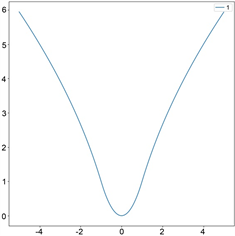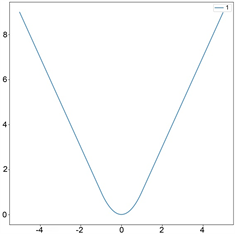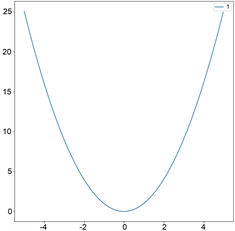$p=0.5$, $\delta =1$ $p=1$, $\delta =1$ $p=2$, $\delta =1$ (a)

$p=0.5$, $\delta =0.1$ $p=0.5$, $\delta =1$ $p=0.5$, $\delta =2$(b)

Figure 2. Diagram of different parameters of p-Huber loss

2.2. 基于p-Huber损失的学习算法

${\mathcal{E}}_{z}\left(f\right)=\frac{1}{m}\underset{i=1}{\overset{m}{\sum }}{\varphi }^{p\text{-Huber}}\left({y}_{i},f\left({x}_{i}\right)\right)$

${f}_{z}=\mathrm{arg}\underset{f\in \mathcal{H}}{\mathrm{min}}\frac{1}{m}\underset{i=1}{\overset{m}{\sum }}{\varphi }^{p\text{-Huber}}\left({y}_{i},f\left({x}_{i}\right)\right)$(2.2)

${f}_{z}=\mathrm{arg}\underset{f\in {\mathcal{H}}_{\mathcal{K}}}{\mathrm{min}}\frac{1}{m}\underset{i=1}{\overset{m}{\sum }}{\varphi }^{p\text{-Huber}}\left({y}_{i},f\left({x}_{i}\right)\right)+\lambda {‖f‖}_{\mathcal{K}}^{2}$(2.3)

${\mathcal{H}}_{\mathcal{K}}=\left\{\underset{i=1}{\overset{m}{\sum }}{\alpha }_{i}\mathcal{K}\left(x,{x}_{i}\right)+b,b\in ℝ,{\alpha }_{i}\in ℝ,i=1,\cdots ,m\right\}$

${\mathcal{K}}_{h}\left({x}_{i},{x}_{j}\right)=\mathrm{exp}\left(-{‖{x}_{i}-{x}_{j}‖}^{2}/{h}^{2}\right)$

3. 仿真实验

3.1. 实验平台及噪声介绍

$\text{noise}:={\tau }_{1}{\epsilon }_{1}+{\tau }_{2}{\epsilon }_{2}^{p}$(3.1)

$\text{Prob}\left({\epsilon }_{2}^{p}=t\right)=\left\{\begin{array}{l}1-p,\text{\hspace{0.17em}}\text{\hspace{0.17em}}t=0,\\ p/2,\text{\hspace{0.17em}}\text{\hspace{0.17em}}\text{\hspace{0.17em}}t=1,\\ p/2,\text{\hspace{0.17em}}\text{\hspace{0.17em}}t=-1.\end{array}$

3.2. 辛格函数下的评估

$f\left(x\right)=\mathrm{sin}\left(\pi x\right)/\left(\pi x\right),x\in \left[-4,4\right]$(3.2)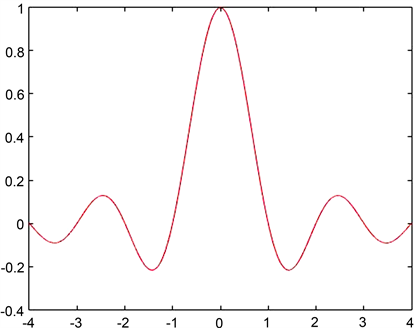Figure 3. One-dimensional Sincfunction graph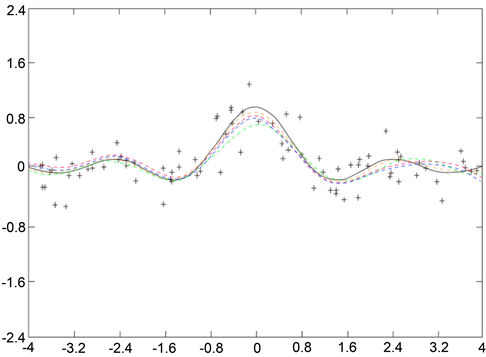Figure 4. The fitting effect diagram of different models to the Sinc function when contaminated by Gaussian noise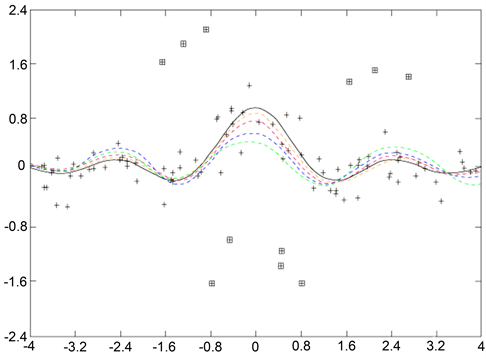Figure 5. The fitting effect diagram of different models to the Sinc function when contaminated by Gaussian noise and outliers

$\text{RSSE}\left(\stackrel{^}{f}\right)=\underset{x\in T}{\sum }{\left(f\left(x\right)-\stackrel{^}{f}\left(x\right)\right)}^{2}/\underset{x\in T}{\sum }{\left(f\left(x\right)-{\stackrel{¯}{f}}_{T}\right)}^{2}$(3.3)Table 1. When δ = 0.7 , different values p predict the result of Sinc functionTable 2. When p = 2.53 , different values δ predict the result of Sinc function

3.3. 弗里德曼基准函数下的评估

${f}_{1}\left(x\right)=10\mathrm{sin}\left(\pi {x}^{1}{x}^{2}\right)+20{\left({x}^{3}-0.5\right)}^{2}+10{x}^{4}+5{x}^{5}$

${f}_{2}\left(x\right)=\sqrt{{\left({x}^{1}\right)}^{2}+{\left({x}^{2}{x}^{3}-1/\left({x}^{2}{x}^{4}\right)\right)}^{2}}$

${f}_{3}\left(x\right)=\mathrm{arctan}\left(1/{x}^{1}\left({x}^{2}{x}^{3}-1/\left({x}^{2}{x}^{4}\right)\right)\right)$

${\left\{{y}_{i}-f\left({x}_{i}\right)\right\}}_{i=1}^{100}$ 都记录下来。对于每个回归模型，其平方误差相对和记录在表3Table 3. The prediction results of different models on Friedman’s benchmark function

3.4. 真实数据集下的评估Table 4. The prediction results of different models on real data

4. 结语

 Davies, P.L. (1993) Aspects of Robust Linear Regression. Annals of Statistics, 21, 1843-1899.
https://doi.org/10.1214/aos/1176349401

 Huber, P.J. (1964) Robust Estimation of a Location Parameter. The Annals of Mathematical Statistics, 35, 73-101.
https://doi.org/10.1214/aoms/1177703732

 Girshick, R. (2015) Fast R-CNN. 2015 IEEE International Conference on Computer Vision (ICCV), Santiago, 7-13 December 2015, 1440-1448.
https://doi.org/10.1109/ICCV.2015.169

 Feng, Y., Huang, X., Shi, L., Yang, Y. and Suykens, J.A.K. (2015) Learning with the Maximum Correntropy Criterion Induced Losses for Regression. Journal of Machine Learning Research, 16, 993-1034.

 Santamaria, I., Pokharel, P.P. and Principe, J.C. (2006) Generalized Correlation Function: Definition, Properties, and Application to Blind Equalization. IEEE Transactions on Signal Processing, 54, 2187-2197.
https://doi.org/10.1109/TSP.2006.872524

 Aronszajn, N. (1950) Theory of Reproducing Kernels. Transaction of the AmericanMathematical Society, 68, 337-404.
https://doi.org/10.1090/S0002-9947-1950-0051437-7

 Zhang, H. and Zhang, J. (2012) Regularized Learning in Banach Spaces as an Optimization Problem: Representer Theorems. Journal of Global Optimization, 48, 1-16.

 Gearhart, W.B. and Schulz, H.S. (1990) The Function Sinx/x. The College Mathematics Journal, 21, 90-99.
https://doi.org/10.1080/07468342.1990.11973290

 Stenger, F. (1981) Numerical Methods Based on the Whittaker Cardinal or Sinc Functions. SIAM Review, 23, 165-224.
https://doi.org/10.1137/1023037

 Friedman, J.H. (1991) Multivariate Adaptive Regression Splines. The Annals of Statistics, 19, 1-67.
https://doi.org/10.1214/aos/1176347963

Top